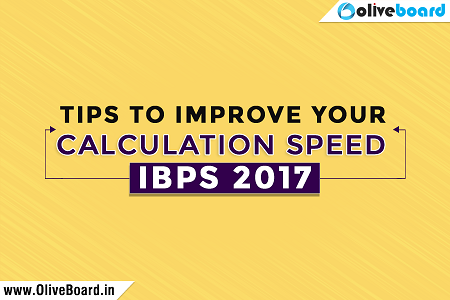IBPS 2017 Exams – How to improve your calculation speed?

IBPS Exams are around the corner. While the first two slots of IBPS PO Prelims were conducted last weekend and the second two slots are scheduled on the coming weekend, IBPS clerk exam is scheduled on the month of December. Here are few tips to improve your calculation speed; this will be helpful for all competitive exams. These tips are brought to you by Oliveboard, an online exam preparation platform for banking and government jobs.Tips to improve calculation speed

1. Memorize multiplication tables up to 20, squares up to 30, cubes up to 20 and fraction tables
2. Get all basics clear and study concepts in order as many are interconnected
3. Include calculations in your everyday life to improve your skills. For example, read statistics in a company’s balance sheet published in a newspaper
4. Practice every day. This will help you identify pattern and thus you can develop your own tricks
5. Maintain notes of important quant formulas for revision
6. Attempt as many mock tests you can to improve your speed and accuracy
7. Analyse every mock to identify your weaker topics and work harder on those
8. Understand few vedic math tricks for faster calculation
9. Keep important theorems and formulas handy to refer again and again during practice
10. Practice mental math to avoid wasting time on rough work during exam

Few Tips & Tricks that will come handy

Multiplying a number by 9

Example: 143 x 9

= 143 x (10-1)

= 1430 – 143

= 1287

Multiplying a 2-digit number by a 2-digit number

Example: 29, 53

9×3=27 (Write 7 and 2 is carried over)

2×3+9×5+2 (carried over) =53 (Write 3 and 5 is carried over)

2×5+5 (carried over) = 15 (write 15)

= 1537

Multiplying a 3-digit number by a 3-digit number

Example: 123, 456

3×6=18 (Write 8 and 1 is carried over)

2×6+3×5+1 (carried over) =28 (Write 8 and 2 carried over)

1×6+3×4+2×5+2 (carried over) =30 (Write 0 and 3 is carried over)

1×5+2×4+3 (carried over) =16 (Write 6 and 1 is carried over)

1×4+1 (carried over) =5 (Write 5)

= 56088

Square of numbers ending in 5 (Example numbers: 65 X 65)

1. Multiply the first number with the next number (6×7 = 42) and this becomes the first part of the answer
2. 25 (5×5) is the second part of the answer